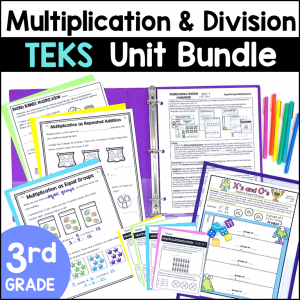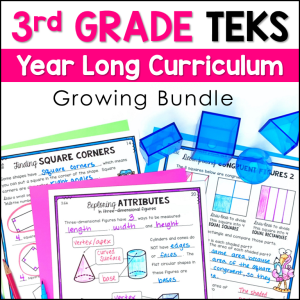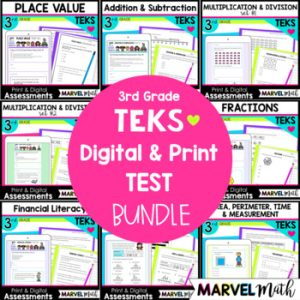Save 10% on your first order with code EXTRA10

# 3rd Grade Multiplication & Division Tests – Set 1 – Digital and Print

\$4.00\$29.99\$151.25\$18.00
SKU: 7200246 Categories: ,

## Description

This resource includes 2 ten question Assessments that cover the Multiplication and Division TEKS. The questions are STAAR formatted. Test questions cover arrays, repeated addition, number lines, missing factors or products, finding odd and even numbers and solving word problems. The assessments are in 2 formats: printable PDF and digital, self-grading Google Forms.

The assessments are created to give you meaningful feedback on student progress with Multiplication Content. There are more questions covering the Readiness Standards than the Supporting Standards, as the Readiness Standards are identified as the most critical content for success in subsequent grade levels. Readiness Standards also comprise 2/3 of the STAAR test.

These tests were created with the new STAAR test questions guidelines in mind, and include drop down, multi-select, free response and multiple choice questions.

I have divided the Multiplication and Division Tests into 2 sets. This resource is Multiplication and Division Test Set #1. Set 1 covers the basics of multiplication and division and includes all supporting standards. Set 2 covers more advanced topics such as multi-step word problems, 2×1 multiplication, and tables to represent real-word relationships.

What’s Included?

• 2 Assessments (Each Test is 10 questions)
• Printable versions of both Assessments
• Color Coded Teacher Key with TEKS identified for each Question

Topics include:

• One-step word problems
• Arrays
• Representing Multiplication with Repeated Addition, Skip Counting, Jumps on a Number Line and arrays
• Using Multiplication facts to solve Division problems
• Division of objects into Equal Groups, or taking out Equal Shares
• Odd and Even Numbers
• Describing Multiplication as a Comparison
• Finding Missing Factors and Products in Multiplication and Division equations

These tests coordinate with the content covered in this set of Task Cards, and these resources work great together!

How Can I Use this Resource?

• Use the 1st test after your Multiplication & Division Unit. Based on the results, do targeted reteach and review. Use the 2nd test to re-assess students.
• Use the 1st test after your Multiplication & Division Unit. Use the 2nd test as part of your STAAR Review.
• Use the 1st test as a pre-test, use the 2nd test as a post-test.

TEKS Alignment:
Supporting Standards

3.4D: Determine the total number of objects when equally sized groups of objects are combined or arranged in arrays up to 10 by 10.

3.4E: Represent multiplication facts by using a variety of approaches such as repeated addition, equal-sized groups, arrays, area models, equal jumps on a number line, and skip counting.

3.4F: Recall facts to multiply up to 10 by 10 with automaticity and recall the corresponding division facts.

3.4H: Determine the number of objects in each group when a set of objects is partitioned into equal shares or a set of objects is shared equally.

3.4I: Determine if a number is even or odd using divisibility rules.

3.4J: Determine a quotient using the relationship between multiplication and division.

3.5C: Describe a multiplication expression as a comparison such as 3 x 24 represents 3 times as much as 24.

3.5D: Determine the unknown whole number in a multiplication or division equation relating three whole numbers when the unknown is either a missing factor or product.